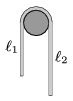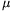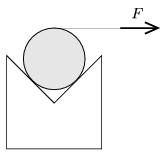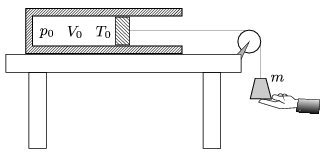Mathematical and Physical Journal
for High Schools
Issued by the MATFUND Foundation
 Already signed up? New to KöMaL?

# KöMaL Problems in Physics, January 2014

Show/hide problems of signs:## Problems with sign 'M'

Deadline expired on February 10, 2014.

M. 338. A horizontal towel rail can hold the towel even if the two parts of the towel hanging on the two sides of the rail are different (see the figure). Measure how the ratio of1/2, when the towel is just about to slip on the rail, depends on the wetness of the towel (the amount of soaked up water).(6 pont)

statistics## Problems with sign 'P'

Deadline expired on February 10, 2014.

P. 4594. Compare the sight of a sparkler on the christmas tree, with the splendour light of the firework show at the new year's eve.

a) In case of the sparkler why can't we observe such expanding, glowing fireballs or fire-wheels (glowing sharpnels which spred out in a plane) as we can see in case of the fireworks?

b) Is it possible to observe elliptical fire-wheels'' during a firework display?

(3 pont)

solution (in Hungarian), statistics

P. 4595. Can the kinetic energy of a dropped ball in Joules be the same as the height measured in metres from which it was dropped?

(3 pont)

solution (in Hungarian), statistics

P. 4596. A cylinder of radius R is lying against the back wall of the bed of a truck, of height h<R. What can the greatest acceleration of the truck be in order that the cylinder does not fall over the wall? (Friction is negligible.)

(4 pont)

solution (in Hungarian), statistics

P. 4597. There is a cylinder in horizontal, wedge shaped, right angled trough as shown in the figure. The cylinder is carefully pulled with an increasing horizontal force by means of a piece of thread wound around it. What happens if the coefficient of friction is

a)=0.5;

b)=0.3?(5 pont)

solution (in Hungarian), statistics

P. 4598. Jill and Jack organise a rolling bicycle race'' along a long enough slope. Their bicycles are alike and none of them pedals the bicycle. Jill weighs 50 kg, Jack is 100 kg, and the mass of the bicycle is 15 kg. The cross sectional area of Jack is one and a half times as much as that of Jill. Who will reach greater terminal speed?

(4 pont)

solution (in Hungarian), statistics

P. 4599. A two-litre bottle with a hand sprayer is filled with liquid to three-quarters of the bottle. After pumping it, in the bottle a gauge pressure equal to the ambient air pressure can be reached. The volume fraction of the water-air mixture, which is slowly sprayed out, is 1:10. The temperature and the density of the effused air can be approximated with those of the ambient air.

a) Estimate at least how many times the bottle must be pumped, in order to spray out 0.5 litre water?

b) In how many doses can all the liquid be sprayed out of the bottle?

(5 pont)

solution (in Hungarian), statistics

P. 4600. A soap bubble is blown with a relatively short drinking straw of diameter d, and then the bubble is left to deflate through the same straw. In one case when the diameter of the bubble was D the time during which the bubble deflated was 8 seconds. Estimate the time during which a bubble deflates

a) if its diameter is also D, and it is deflated through a wider straw of diameter 2d;

b) through a straw of diameter d, if its greatest diameter was 2D?

(5 pont)

solution (in Hungarian), statistics

P. 4601. An easily moveable, thermally insulated piston of negligible mass confines a sample of air of volume V0=8 litre, at a temperature of T0=300 K, into a thermally insulated cylinder of cross section A=1dm2. The cylinder is fixed to a horizontal table. The pressure of the confined air is the same as the atmospheric pressure p0=105 Pa. A piece of thread is attached to the centre of the piston, it lies in the line of the axis of the cylinder and leads through a pulley. A weight of mass m=25 kg is attached to the other end of the thread as shown in the figure. Holding the weight, any part of the thread is straight, but loose.The weight is released without any push. To an accuracy of 1% determine the

a) temperature of the air in the cylinder, when the weight is at its lowermost position;

b) the acceleration of the piston at the lowermost position of the weight;

c) the greatest speed of the piston during the process. (The mass of the thread and the pulley and friction are negligible.)

(5 pont)

solution (in Hungarian), statistics

P. 4602. Two lenses of optical power of 10 dioptres are placed such that their principal axis coincide. There is a well-illuminated object 12-cm from one of them - the other lens is on the other part of the lens. The other lens forms a virtual, 50-times magnified image of the object. By what distance does this lens need to be moved in order to create a 50-times magnified image on a screen?

(4 pont)

solution (in Hungarian), statistics

P. 4603. Calculate the equivalent resistance of the resistor chain shown in the figure between the points a) A and B; b) A and C the resistor chain is infinite in both directions and each resistor has a resistance of R.(5 pont)

solution (in Hungarian), statistics

P. 4604. There are two big, same size, uncharged metal sheets in the same plane, lying parallel and very close to each other. The sheets are connected through a piece of wire, and then a pointlike charge Q is placed next to them as shown in the figure. What will the amount of charge on each metal sheet be?(6 pont)

solution (in Hungarian), statistics